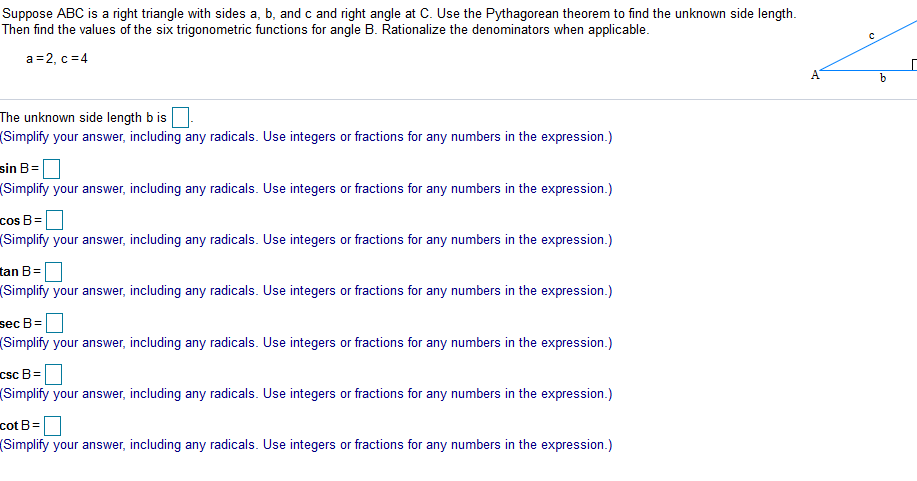# In any angle ABC prove tht the perimeter = a/Sin A(Sin A + Sin B + Sin C) Start with perimeter = a+b+c but b= aSinB/sinA and c= aSinC/SinA and if you dont remember, a=a=a*SinA/ SinA

In any angle ABC prove tht the perimeter = a/Sin A(Sin A + Sin B + Sin C)

but b= aSinB/sinA and
c= aSinC/SinA

and if you dont remember, a=a=a*SinA/
SinA

This Homework Help Question: "In any angle ABC prove tht the perimeter = a/Sin A(Sin A + Sin B + Sin C) Start with perimeter = a+b+c but b= aSinB/sinA and c= aSinC/SinA and if you dont remember, a=a=a*SinA/ SinA" No answers yet.

We need 3 more requests to produce the answer to this homework help question. Share with your friends to get the answer faster!

0 /3 have requested the answer to this homework help question.

Once 3 people have made a request, the answer to this question will be available in 1-2 days.
All students who have requested the answer will be notified once they are available.
##### Add Answer of: In any angle ABC prove tht the perimeter = a/Sin A(Sin A + Sin B + Sin C) Start with perimeter = a+b+c but b= aSinB/sinA and c= aSinC/SinA and if you dont remember, a=a=a*SinA/ SinA
Similar Homework Help Questions
• ### if A+B+C=180degrees, prove that 2sin(B+C)sinA=1-cos2A

if A+B+C=180degrees, prove that 2sin(B+C)sinA=1-cos2A

• ### in triangle abc ad is bisector of angle a and angle b is twice of angle c prove that angle bac is equal to 72

in triangle abc ad is bisector of angle a and angle b is twice of angle c prove that angle bac is equal to 72

• ### A, B, and C are 3 angles in triangle ABC then sin(A+B)=?

(a) sinA (b)sinB (c)sinC (d)cosC (e) -sin(A+B)

• ### I don't know the physics behind Snell's Law, but I can do the math Snell's Law says sin(angle of incidence)/sin(angle of reflection) = refractive index since you know the two angles

I don't know the physics behind Snell's Law, but I can do the math Snell's Law says sin(angle of incidence)/sin(angle of reflection) = refractive index since you know the two angles... sin45/sin27 = refractive index =1.5575365 so for an angle of refection of 25º sin(incidence angle)/sin25º=1.5575.. sin(incidence angle)=sin25(1.55..) =.6582433 so angle of incidence = arcsin(.658..) =41.166º That's just the application of Snell's, that part I've done. What I need is: sin(a)/sin(a-25) = RII would solve it by iteration. There is...

• ### (Using ABC, find the following: a) The length of side AC b) The perimeter of ABC c) The area of ABC c=90' AB= 13 in

(Using ABC, find the following: a) The length of side AC b) The perimeter of ABC c) The area of ABC c=90' AB= 13 in. CB= 12 in.

• ### Suppose ABC is a right triangle with sides a, b, and c and right angle at...Suppose ABC is a right triangle with sides a, b, and c and right angle at C. Use the Pythagorean theorem to find the unknown side length. Then find the values of the six trigonometric functions for angle B. Rationalize the denominators when applicable. с a=2, c = 4 b The unknown side length bis (Simplify your answer, including any radicals. Use integers or fractions for any numbers in the expression.) sin B= (Simplify your answer, including any radicals. Use...

• ### Given: angle A is congruent to angle B angle B is congruent to angle C Prove: angle A is congruent to angle C

Given: angle A is congruent to angle B angle B is congruent to angle C Prove: angle A is congruent to angle C

• ### in triangle abc angle b is 5x angle a and c is 16 degree less than 4 times angle a the size of angle a b c in decimal is

in triangle abc angle b is 5x angle a and c is 16 degree less than 4 times angle a the size of angle a b c in decimal is

• ### In a triangle ABC, angle B is three times angle A and angle C is 1 less than 6 times angle A

In a triangle ABC, angle B is three times angle A and angle C is 1 less than 6 times angle A. Find the size of angle A, B, C

• ### if you have line with points dabe and you have triangle acb sitting on top of the line you are given angle dac is congruent to angle ebc prove triangle abc is isosceles

if you have line with points dabe and you have triangle acb sitting on top of the line you are given angle dac is congruent to angle ebc prove triangle abc is isosceles

Free Homework App